﻿ Tidal Methodology - BEM Analysis >

# Methodology for a Decision Support Tool for a Tidal Stream Device

MSc Sustainable Engineering: Offshore Renewable Energy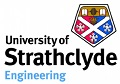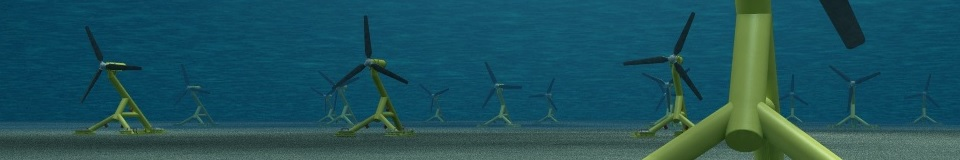This tool performs a blade design optimization and obtains the Annual Energy Production for a defined turbine using a Genetic Algorithm applied to Blade Element Momentum (BEM) theory.

Once the previous tools have defined the tidal resource and the physical constraints of the site, a study of the Power Generation is performed to estimate the electrical power that can be extracted from a specific turbine with a selected material.

The Blade Element Momentum (BEM) analysis is implemented in HARP_Opt, a freeware tool developed by the National Renewable Energy Laboratory (NREL).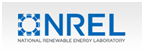NREL Simulators

HARP_Opt is based on different Matlab files that are combined together with the WT_Perf Blade Element Momentum code of the National Renewable Energy Laboratory to obtain the power generation of Horizontal Axis Tidal Turbines.

### Inputs

In this section the Inputs of the Tool are presented for the analysis of a 22 [m] Aluminium turbine in the Mull of Kintyre:

Turbine Configuration
•   Control Configuration
o   Fixed or Variable Speed Control
o   Fixed or Variable Blade Pitch Control
•   Rated Mechanical Power of the Rotor [kW]
•   Rotor Diameter [m]
•   Hub Diameter [m]
•   Pre Cone [deg]
•   Shaft Tilt [deg]
•   Minimum Allowable Rotor Speed [m/s]
•   Maximum Allowable Rotor Speed [m/s]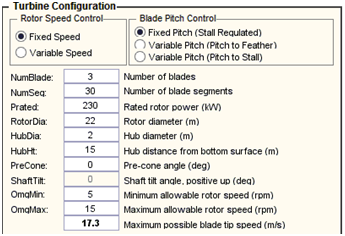Turbine configuration interface

Hydrokinetic Turbine Configuration
•   Water Vapour Pressure [Pa]
•   Air Atmospheric Pressure [Pa]
•   Water Depth [m]
•   Cavitation Safety Factor [-]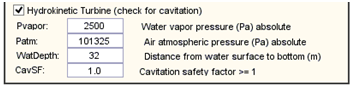Hydrokinetic Turbine configuration interface

Fluid Properties
•   Fluid Density [kg/m3]
•   Kinematic Fluid Viscosity [m2/s]
•   Minimum Flow Speed [m/s]
•   Maximum Flow Speed [m/s]
•   Flow Speed Increment [m/s]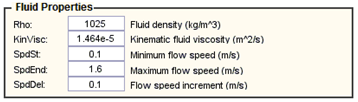Fluid Properties configuration interface

WT_Perf Algorithm Configuration
•   Prandtl Tip-Loss Algorithm Usage
•   Prandtl Hub-Loss Algorithm Usage
•   Rotational Wake Effects Usage
•   WT_Perf PROP Algorythm Usage
•   Drag Term Usage in Axial Induction Calculation
•   Drag Term Usage in Tangential Induction Calculation
•   Maximum Number of Iterations for Induction Factors [-]
•   Error Tolerance for Induction Factors [-]
•   Lift Selig-Du Correction Usage
•   Drag Eggers Correction Usage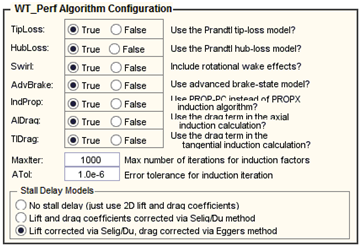WT_Perf Algorithm configuration interface

Optimization Objectives
•   Efficiency or Annual Energy Production (AEP) Optimization
•   Flow Distribution
o   Rayleigh Distribution
o   Weibull Distribution
o   User-Defined Distribution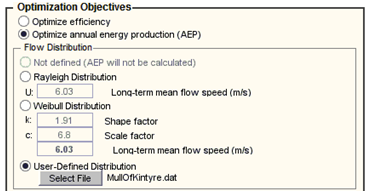Optimization Objectives configuration interface

Structural Optimization
• Young Modulus [GPa]
• Material Density [kg/m3]
• Maximum Allowable Strain [micro-strain]
• Bending Moments Safety Factor [-]
• Minimum Shell Thickness [mm]
• Shell Thickness Increment [mm]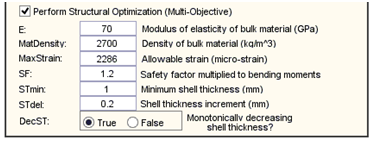Structural configuration interface

•   Pre-Twist and Chord Distributions
o   Twist Lower Bound [m]
o   Twist Upper Bound [m]
o   Chord Lower Bound [m]
o   Chord Upper Bound [m]
•   Hydrofoil Distribution
o   Blade Family (FFA, NREL, NACA…)
o   Thickness % distribution along the chord [%]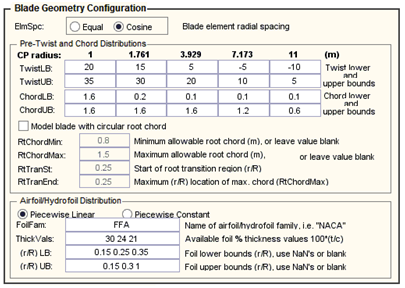Genetic Algorithm Configuration
• Number of Individuals per Generation [-]
• Number of Elite Individuals per Generation [-]
• Fraction of Population to Remain on Pareto Front [-]
• Fraction of Individuals Created by Crossover [-]
• Maximum Number of Generations for Genetic Algorithm Iterations [-]
• Error Tolerance for the Genetic Algorithm Fitness Value [-]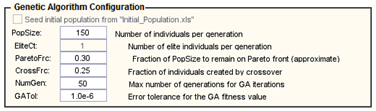Genetic Algorithm configuration interface

### Outputs

The outputs from the tool are a set of different optimum blade designs obtained with the Genetic Algorithm. The number of designs will be equal to: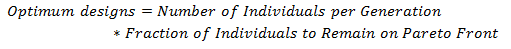Genetic Algorithm configuration interface

For each one of the design the following outputs are obtained:

• Rotor Power [kW] and Power Coefficient [-]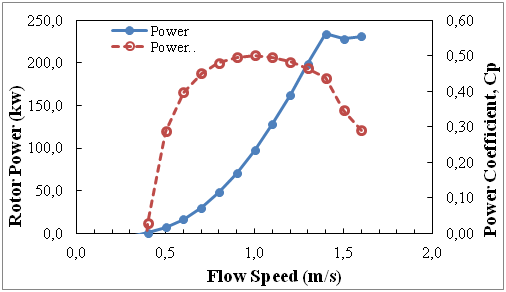Rotor Power [kW] and Power Coefficient [-]

• Rotor Flap Moment [kN-m]
• Torque [kN-m]
• Chord [m] and Pre-Twist [deg]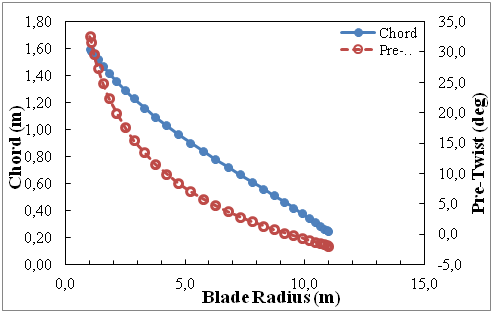Rotor Power [kW] and Power Coefficient [-]

• Thickness [%] and Dimensional Thickness [m]
• Shell Thickness [mm] and Mass per unit span [kg/m]
• Strain [micro-strain]
• Stress [MPa]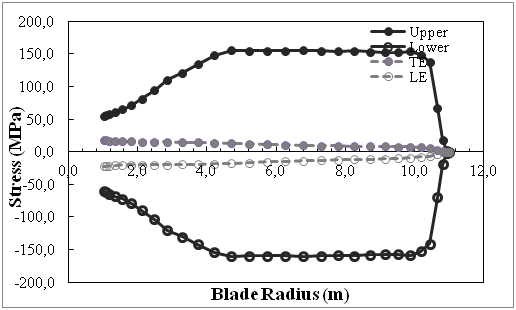Stress [MPa]

• Area Moment of Inertia [m4]
• Moment [kN-m]

### Methodology

This tool is based in over 50,000 lines of Matlab code and the explanation of all the theory behind it is well beyond the scope of this project. All the lines of the code have been read and understood (and in some cases modified) for a correct usage of the tool. A good explanation of the methodology can be found in the User Guide of FAST, a Blade Element Momentum tool of National Renewable Energy Laboratory (NREL):

User Guide of FAST (NREL)

Momentum Theory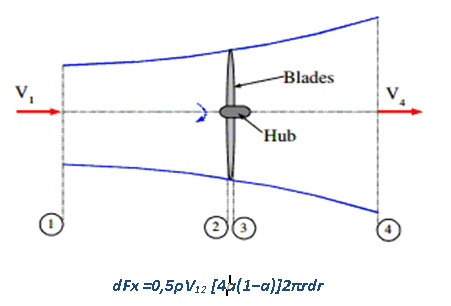Axial Force – Bernoulli Equation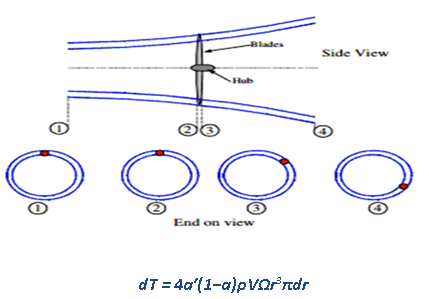Rotating Angular Stream Tube – Angular Moment Conservation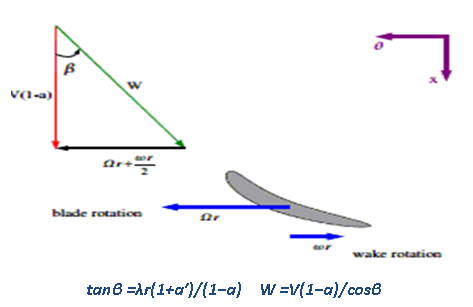Relative Force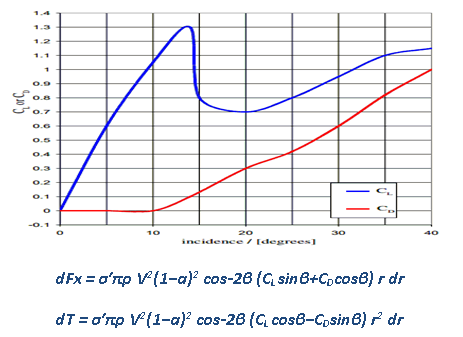Genetic Algorithm

Genetic Algorithms are used to find the best possible solution when it is not known in advance which one the optimum solution is by using the principles of the biological evolution.

It is used to iteratively modify an Initial Population by different processes of Selection, Recombination and Mutation on which the superior individuals have more probabilities to survive. Therefore, after a certain number of iterations the existing population will be optimal.

MATLAB Genetic Algorithm is used in HARP_Opt to optimize the blade properties against Annual Energy Production obtained from WT_Perf Blade Element Momentum theory code.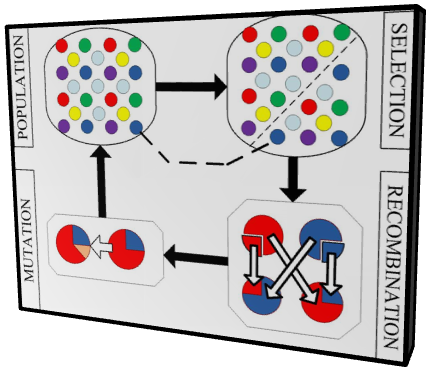Genetic Algorithm: (LUREC – Lancaster University Renewable Energy Group)

References:
➙    Ingram, Grant: Wind Turbine Blade Analysis using the Blade Element Momentum Method. October 2011